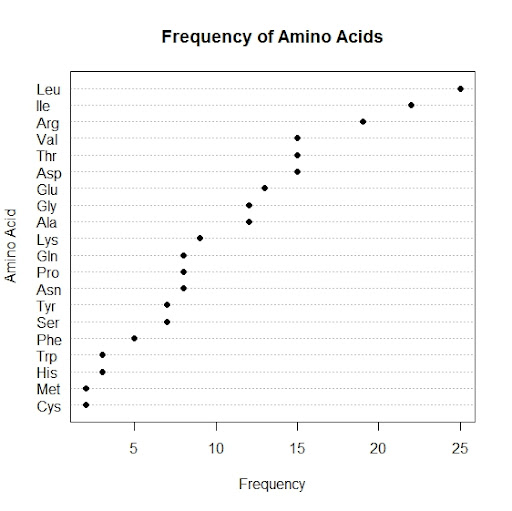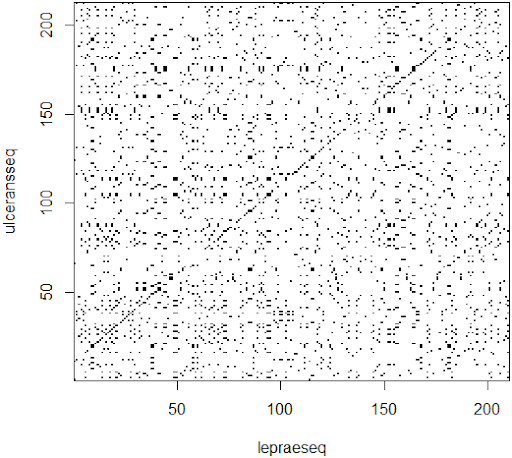## Posts

Showing posts from June, 2013

### Frequency Plot of Protein Sequences using RA frequency plot is a graphical data analysis technique for summarizing the distributional information of a variable. The response variable is divided into equal sized intervals (or bins). The number of occurrences of the response variable is calculated for each bin. In this tutorial, the number of occurrences of each amino acids in the protein sequence (response variable) is calculated and sorted in ascending order. The frequency plot then consists of: Vertical Axis = Amino acids Horizontal Axis = Frequencies of the amino acids There are 4 types of frequency plots: Frequency plot (absolute counts); Relative frequency plot (convert counts to proportions); Cumulative frequency plot; Cumulative relative frequency plot. The frequency plot and the histogram have the same information except the frequency plot has lines connecting the frequency values, whereas the histogram has bars at the frequency values. Frequency plot using R In this tutorial, the programming language R and

### DotPlot for Protein Sequences using RDotplot is the visual representation of the similarity between two protein or nucleotide sequences. Dotplot was introduced by Gibbs and McIntyre in 1970 and are two-dimensional matrices that have the sequences of the proteins being compared along the vertical ( y ) and horizontal ( x ) axes. Individual cells in the matrix can be shaded black if residues are identical, so that matching sequence segments appear as runs of diagonal lines across the matrix. The closeness of the sequences in similarity will determine how close the diagonal line is to what a graph showing a curve demonstrating a direct relationship is. This relationship is affected by certain sequence features such as frame shifts , direct repeats , and inverted repeats . Frame shifts include insertions, deletions, and mutations. The presence of one of these features, or the presence of multiple features, will cause for multiple lines to be plotted in a various possibility of configurations, depending on the features pre